## General Question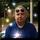# Does anyone know a mathematical formula to calculate the surface area of bubbles in a sink or bathtub of suds?

Asked by Yellowdog (8217) 1 month ago

Observing members: 0Composing members: 0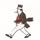Too many variables when it comes to the size and quantity of the bubbles.

stanleybmanly (20240)“Great Answer” (2) Flag as…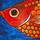If you call the width of the bath or sink R then very roughly I guess the area would be 2piR squared.

flutherother (29801)“Great Answer” (2) Flag as…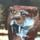It would be a fractal equation, but I’m not sure how that works.

Patty_Melt (13431)“Great Answer” (2) Flag as…@flutherother. No. You have to determine the surface area of the individual bubbles (4 pi r squared) Then add the totals. This is another one of those “angels on the head of a pin questions” As asked? There isn’t enough information provided for the question to be sensibly understood. For example, there is no standard size of a bubble, there are bubbles that meld into others, and there is no determining how high the froth of bubbles will extend above the surface. The question amounts to a pointless riddle of nothing but unknowns.

stanleybmanly (20240)“Great Answer” (4) Flag as…@stanleybmanly The bubbles would not be spherical as some would sit on the bath water or on top of other bubbles. That is why I divided by two. It is a wild approximation to a question similar to “how long is a piece of string?”

flutherother (29801)“Great Answer” (4) Flag as…That’s exactly my point. The question asks the total surface area of a “surface” where the individual bubbles can be of any size or quantity. The dimensions of the container are useless because the height of the bubbles above the surface must vary a great deal from point to point. Consider how you would determine the total surface area of marbles in the tub or sink if the marbles were of random size. The best you might do is to approach a limit to the combined area, with the smallest surface area assigned to a single giant marble occupying the tub and the upper limit dependent on how small you might be able to make a marble.

stanleybmanly (20240)“Great Answer” (3) Flag as…The bubbles would occupy 74% of the total volume Divide by the volume of the bubbles, 4/3 pi r**3, and multiply by the surface area of a bubble, 4 pi r**2 to get .74*3/r V.

LostInParadise (26115)“Great Answer” (2) Flag as…@LostInParadise The bubbles in my experience aren’t of equal size and would also tend to stick together with shared surfaces.

flutherother (29801)“Great Answer” (2) Flag as…It should make for a good first approximation using an estimate of average bubble size.

LostInParadise (26115)“Great Answer” (3) Flag as…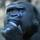@LostInParadise Would you use average bubble size, or would it make sense to do some kind of area under the curve equation where we assume there will be bigger bubbles and smaller bubbles and the smaller ones will occupy the gaps between the bigger ones? Perhaps there’s a formula for packing ever decreasing radii of spheres into a cube, and one could use that? I would guess this would approach some limit, since we know the total volume of the cube.

I’m just guessing because I think the math is more advanced than my skills.

gorillapaws (23607)“Great Answer” (2) Flag as…The math is certainly beyond my skills, particularly as flutherother has pointed out, the suds or bubbles are not all spherical. Nor can they possibly be said to in any way conform to or even resemble anything as efficiently stacked as a lattice or regular sorting of any kind. The formula for the volume of a sphere WOULD do the trick IF the suds and bubbles were all spherical AND “packed” in consistent order or density. If anyone can demonstrate that this is the case, I will be more than surprised.

stanleybmanly (20240)“Great Answer” (2) Flag as…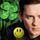I’d weigh the soap, assume a density near water, 1.0. That would give me a total volume. I’d then make the assumption that the bubble walls were of a sheet 2 wavelengths of light thick – call it 1200 nm. That would be thiker than actual but might account for edges. I’d then divide the volume by 1200nm to get the largest possible area of the bubbles.

LuckyGuy (37076)“Great Answer” (5) Flag as…@LostInParadise Your solution of course works for the marbles IF you are able to apply the brute force method of determining the area of individual marbles, but the bubbles including those which have merged with plane surfaces at their intersections, the variety in size and geometry is the visual demonstration of the chaotic distribution of surfactant per bubble which is impossible to formualize.

stanleybmanly (20240)“Great Answer” (2) Flag as…or